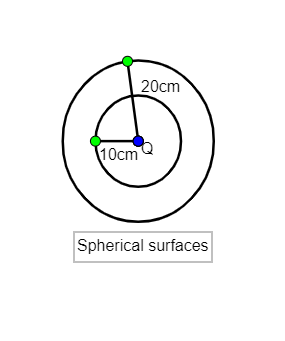QuestionAnswers

# Electric charges are distributed in a small volume. The flux of the electric field through a spherical surface of radius 10cm surrounding the total charge is 25 V-m. The flux over a concentric sphere of radius 20 cm will be:$A.$ 25V-m$B.$ 50V-m$C.$ 100V-m$D.$ 200V-m

Hint: The flux through a surface does not depend on its shape and size; it only depends upon the charge enclosed by the sphere of radius 10 cm and the sphere of radius 20 cm is same so the flux through them will also be the same.

Values given to us are flux through a surface of 10cm is ${{\phi }_{10}}$=25 V-m
$Q_{en}^{10}$ is charge enclosed within a radius of 10cm
$Q_{en}^{20}$is the charge enclosed within a radius of 20cm
${{\phi }_{20}}$ is the flux through a surface of 20cmUsing Gauss’s law, ${{\phi }_{10}}=\dfrac{Q_{en}^{10}}{{{\in }_{0}}}$
Or $Q_{en}^{10}=25{{\in }_{0}}$
Here for flux of radius 20cm, $Q_{en}^{20}=Q_{en}^{10}=25{{\in }_{0}}$
Thus, ${{\phi }_{20}}=\dfrac{25{{\in }_{0}}}{{{\in }_{0}}}=25$V-m.

So, the correct answer is “Option A”.

Electric field passing through a given area at a particular time is known as electric flux. Electric flux is directly proportional to the number of electric field lines passing through that given area. If the electric field is uniform, the electric flux passing through a surface of vector area S is: $\phi =E\cdot S=EScos\theta$, where E is the magnitude of the electric field having units of V/m, S is the area of the surface, and θ is the angle between the electric field lines and the normal to S. Electric flux is also related to Gauss law. Gauss Law states the net electric flux from a closed surface is equal to $\dfrac{1}{{{\in }_{0}}}$ times the net charge enclosed in that area. There are two forms of the Gauss law; the integral form and the differential form.